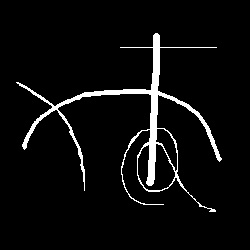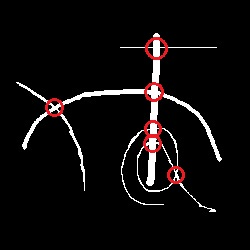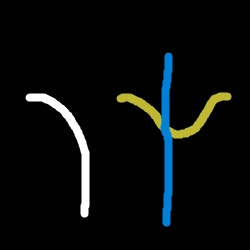# Finding intersections of curves in a binary image

I am trying to find the intersection points in images like the one posted below. The intersections I'm trying to find are circled in red in the image below it.My most succesful approach:

1. Thin the image
2. Perform a Hough transform, peak identify, and back project
3. Identify peaks in the back projection and select those as intersection points
4. If there are too many peaks(I say 4 is too many), go to a smaller section of the image and repeat the above steps

Obviously a large problem here is that the Hough transform for detecting lines isn't supposed to detect curves. I thought zooming in on points of interest might fix this, but it has not. Some curves are just still too sharp, and if you zoom in too far the transform doesn't have enough data to make accurate calculations of where lines are.

I've also tried some local feature detection, such as corner detection, to find the intersections. Local features fail miserably, and there are some images to explain why that is as well (although I am currently toying with another approach using gradients; will update post after I implement it).The white curve isn't an intersection, but the blue and yellow curves obviously form an intersection. The curves, however, have a section of nearly identical local features when converted into a binary image. Any suggestions how to find these intersecting points would be greatly appreciated!

• Are you familiar with curvelets? They might be useful here: curvelet.org
– user20857
May 4, 2017 at 3:42
• I am not. I will have to look into this. Thank you! May 4, 2017 at 14:09
• One way to do this is to thin the image then look at the skeleton, also see this May 20, 2017 at 0:06
• Maybe a supervised learning technique might work well here? May 20, 2017 at 15:54
• I'm also thinking of an "ants marching" type of algorithm. You could design an algorithm, "ants" that try to stay within the lines. Keep track of the paths. Then look for intersections in the path data. May 20, 2017 at 15:56

You can also have a look at detecting crossing with the local Hessian matrix : https://dsp.stackexchange.com/questions/10579/how-hessian-feature-detector-works It can detect "corners" (intersection of 2 lines), but maybe the limitation would be to determined between a sharp curve (like if it goes a 90° angle) and an actual intersection of 2 independant curves.

I am still looking for a better answer, but I found a solution for smooth lines with little to no noise (so still fairly useless). Turns out that when a binary image such as the ones above is thinned, one of two things occurs at intersections: either the connected component is broken, or a pixel in the general area is connected to 2 pixels instead of 1. Solution: modified connected components

//PLEASE NOTE: THE IMAGE MUST BE THINNED FIRST!! Read above paragraph
int width = img.length;
int height = img.length;
int intersections = 0;
for (int i = 1; i < width - 2; i++){
for (int j = 1; j < height - 2; j++){
if (img[i,j] > 50){
//initialize a queue for this connected component
ArrayList<Integer[]> queue = new ArrayList<>();
img[i, j] = 0;
while (!queue.isEmpty()){
Integer[] c = queue.remove(0);
int count = 0;
for (int x = -1; x < 2; x++){
for (int y = -1; y < 2; y++){
if (img[c+ x, c + y] > 50){
queue.add({c + x, c + y});
img[c + x, c + y] = 0
//count the number of pixels connected to this one
count += 1;
}
}
}
if (count > 1){
//if more than 1 pixel was connected, it's at an intersection
intersections += 1;
}
}
}
//add 1 intersection for every connected component found
intersections += 1;
}
}
//correct for the original number of connected components in the image
//minus 1; helps offset against tendency for false negatives
intersections -= original_number_of_connected_components - 1
return intersections / 2;


The above is written in Java to expose the logic, but I implemented it in IDL, so I haven't debugged this (i.e. no promises this code compiles when put inside an actual method. In fact it most definitely won't if you don't pass in an array as an image and the original_number_of_connected_components parameter).

EDIT: This does a horrendous job, as one might expect, on jagged or noisy images. Still looking for a better solution.

EDIT: I have a solution that does an OK job. It will be on GitHub on October 19th, when I become much less busy. It's off on some images that don't thin very well, but is usually in the same ball park, and does not work on self intersecting images at the moment. This answer will completely change on the 19th, and unless I receive a fairly fool proof solution by then, I will revamp this answer and accept it as correct.